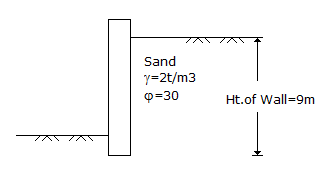# Civil Engineering - UPSC Civil Service Exam Questions

26.

Active earth pressure per metre length on the retaining wall with a smooth vertical back as shown in the given figure will beA. 81 t B. 27 t C. 2 t D. 1 t

Explanation:

No answer description available for this question. Let us discuss.

27.

Which of the following rules are used in choosing the repeating variables in dimensional analysis :
1. Repeating variables should include the dependent variables
2. Repeating variables should contain all primary units used in describing the variables in the problem
3. Repeating variables should combine among themselves.
4. Repeating variables should not contain the dependent variables.
Select the correct answer using the codes given below :

 A. 1 and 2 B. 2 and 3 C. 2 and 4 D. 3 and 4

Explanation:

No answer description available for this question. Let us discuss.

28.

The proportion of second moment of area about the centroidal axis to second moment of area about the base of a rectangle will be

 A. 1/4 B. 1/2 C. 2 D. 3

Explanation:

No answer description available for this question. Let us discuss.

29.

Mean precipitation over an area is best obtained from gauged amounts by

 A. arithmetic mean method B. Thiessen method C. linearly interpolated isohyetal method D. orographically weighted isohyetal method

Explanation:

No answer description available for this question. Let us discuss.

30.

Loses deposits are formed by

 A. physical disintegration of rock B. constant blowing of wind from the same direction C. vertical deposition of glacial till D. chemical weathering of residual deposits.